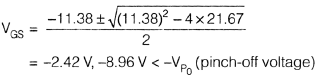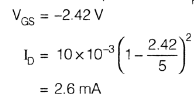Courses

# Electronic Devices - MCQ Test - 2

## 20 Questions MCQ Test GATE ECE (Electronics) 2022 Mock Test Series | Electronic Devices - MCQ Test - 2

Description
This mock test of Electronic Devices - MCQ Test - 2 for Electronics and Communication Engineering (ECE) helps you for every Electronics and Communication Engineering (ECE) entrance exam. This contains 20 Multiple Choice Questions for Electronics and Communication Engineering (ECE) Electronic Devices - MCQ Test - 2 (mcq) to study with solutions a complete question bank. The solved questions answers in this Electronic Devices - MCQ Test - 2 quiz give you a good mix of easy questions and tough questions. Electronics and Communication Engineering (ECE) students definitely take this Electronic Devices - MCQ Test - 2 exercise for a better result in the exam. You can find other Electronic Devices - MCQ Test - 2 extra questions, long questions & short questions for Electronics and Communication Engineering (ECE) on EduRev as well by searching above.
QUESTION: 1

### For a P-channel Ge JFET, it is having doping concentration of Na = 1.77 × 1015 cm−3 and Nd = 3.53 × 1018 per cm3, If channel thickness is a = 1 µ met. (Given Relative permittivity of Ge = 16 at T = 300° K)  Q. What is value of internal pinch-off voltage?

Solution: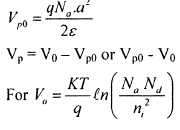QUESTION: 2

### For a P-channel Ge JFET, it is having doping concentration of Na = 1.77 × 1015 cm−3 and Nd = 3.53 × 1018 per cm3, If channel thickness is a = 1 µ met. (Given Relative permittivity of Ge = 16 at T = 300° K)  Q. What is value of pinch-off voltage?

Solution:QUESTION: 3

### If saturation currents of 2 diodes are 1 µA and 2 µA. If break down voltages of diode are same and are equal to 100 volt, what is value of current in D1.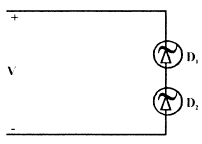Q. If V = 90 volt

Solution:
QUESTION: 4

If saturation currents of 2 diodes are 1 µA and 2 µA.
If break down voltages of diode are same and are equal to 100 volt, what is value of current in D1.Q.

If V = 110 volt

Solution:
QUESTION: 5

For Si transistor, if β ≥ 30 and ICBO = 10 nA  The minimum value of R, for transistor to remain in active region for vi = 12 volt, is __________ kΩ.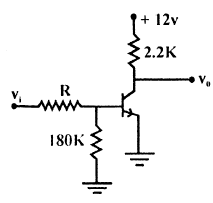Solution:
QUESTION: 6

A sample of silicon (uniformly doped n-type) at T = 300° K has the electron concentration varying linearly with distance as shown in the figure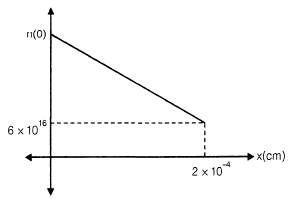The diffusion current is found to be −1120 A/cm2. If the diffusion constant Dn = 35 cm2/s, the electron concentration at x = 0 is

Solution: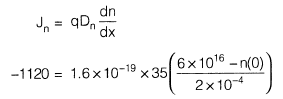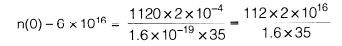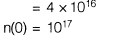QUESTION: 7

In a semiconductor sample,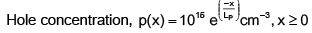where LP (hole diffusion length) = 4.8 × 10−4 cm

Hole diffusion coefficient DP = 20 cm2/s

The hole diffusion current density at x = 0 is

Solution: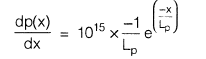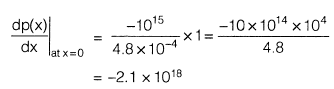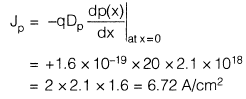QUESTION: 8

In the plot of log I vs V for a semiconductor Ge diode, the slope at room temperature is _________. Assume V >> VT and room temperature 27°C.

Solution: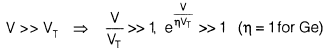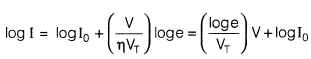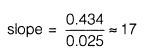QUESTION: 9

Consider a p-type semiconductor which is lightly doped i.e. condition of p >> n is not valid. p, n, ni are holes, electrons and intrinsic carrier concentration respectively. Then 2n + NA is ___________ if NA is immobile acceptor ions concentration

Solution:

According to charge neutrality,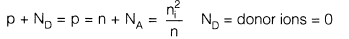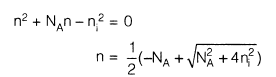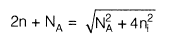QUESTION: 10

Consider the following network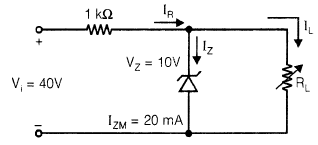VL is to be maintained at 10 V.
The correct representation of VL versus RL is

Solution: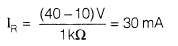RLmin to turn-on the zener diode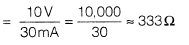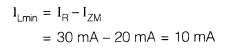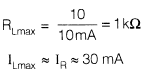QUESTION: 11

Consider the following sentences in respect of LEDs (Light emitting diodes) and semiconductor laser diode.
S1 :  Only direct band gap type semiconductors are suitable for fabrication of LEDs.
S2 : Both direct band gap type and indirect band gap type semiconductors are suitable for fabrication of semiconductor laser diode.
Choose the best alternative

Solution:

For the fabrication of semiconductor laser diode, direct band gap type semiconductor is required

QUESTION: 12

If individual JFET has IDSS = 5 mA and pinch-off voltage  VP0 = 3V ,  then current iD for VGS = − 0.9 V is __________ mA.

Solution: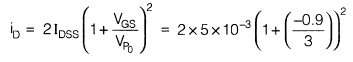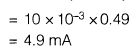QUESTION: 13

What are the states of three ideal diodes of circuit shown below: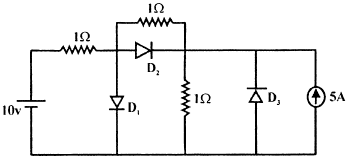Solution:
QUESTION: 14

For BJT, circuit shown assume that β of transistor is very large, if here Si transistor is used, then transistor will operate in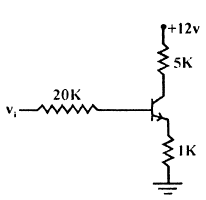Solution:

If β is large then transistor will be in S.R

QUESTION: 15

Consider the following circuit: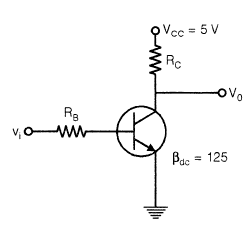Q.

If vi is as shown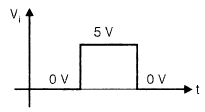then RB and RC for the circuit concerned can be _________ and _________ respectively, if ICsat = 6 mA. Assume the transistor inverter operation

Solution: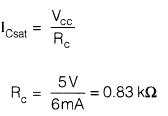Just at onset of saturation,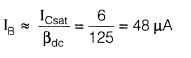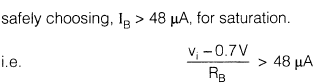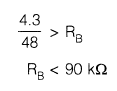QUESTION: 16

Consider the following circuit:Q.

The V0 shall be represented by

Solution:

Transistor is switch in inverter operation.

QUESTION: 17

A BJT having β = 125 is biased at a dc collector current of 1.23 mA. The values of gm, re and rπ at the bias point are: (Assume temperature of operation = 25°C).

Solution: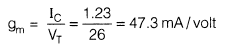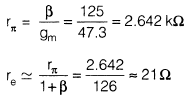QUESTION: 18

A properly biased JFET may be shown by one of the following figures.Choose the correct alternative: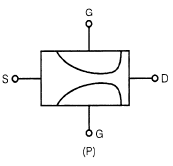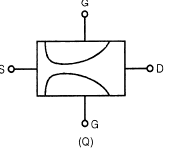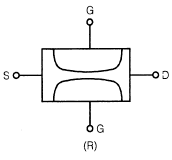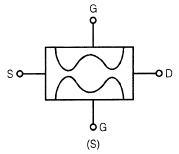Solution:

Because of the more reverse biased drain end, the depletion width is thinner in drain side.

QUESTION: 19

Shown below is the n-channel JFET in voltage divider bias arrangement.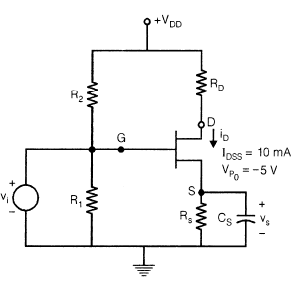VDD = 12 V
RS = RD = 1.8 kΩ

R1 = 100 kΩ

R2 = 400 kΩ

Q.

Gate to source voltage (VGS) is given by the quadratic equation

V2GS + PVGS + Q = 0
P and Q are respectively

Solution: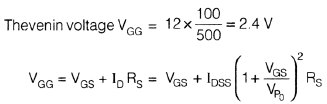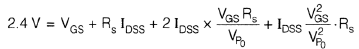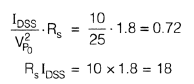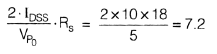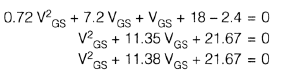QUESTION: 20

Shown below is the n-channel JFET in voltage divider bias arrangement.VDD = 12 V
RS = RD = 1.8 kΩ

R1 = 100 kΩ

R2 = 400 kΩ

Q.

The drain current iD is

Solution:

Solving VGS from the obtained quadratic equation